﻿ Common Core Standards for Math - KindergartenAdaptive Worksheets

Helping Every Child to Succeed

toll-free (888) 777-0876
fax (888) 777-0875

First Grade

## Extend the Counting Sequence

1.NBT.1. Count to 120, starting at any number less than 120. In this range, read and write numerals and represent a number of objects with a written numeral.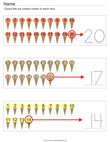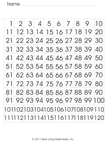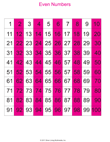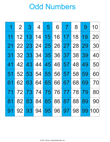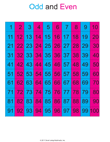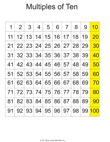## Understand Place Value

1.NBT.2. Understand that the two digits of a two-digit number represent amounts of tens and ones. Understand the following as special cases:
• 10 can be thought of as a bundle of ten ones — called a “ten.”
• The numbers from 11 to 19 are composed of a ten and one, two, three, four, five, six, seven, eight, or nine ones.
• The numbers 10, 20, 30, 40, 50, 60, 70, 80, 90 refer to one, two, three, four, five, six, seven, eight, or nine tens (and 0 ones).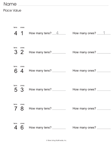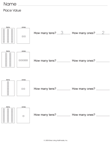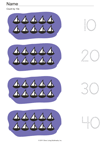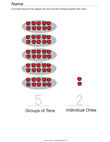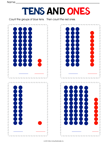1.NBT.3. Compare two two-digit numbers based on meanings of the tens and ones digits, recording the results of comparisons with the symbols >, =, and <.

## Use place value understanding and properties of operations to add and subtract

• 1.NBT.4. Add within 100, including adding a two-digit number and a one-digit number, and adding a two-digit number and a multiple of 10, using concrete models or drawings and strategies based on place value, properties of operations, and/or the relationship between addition and subtraction; relate the strategy to a written method and explain the reasoning used. Understand that in adding two-digit numbers, one adds tens and tens, ones and ones; and sometimes it is necessary to compose a ten.
• 1.NBT.5. Given a two-digit number, mentally find 10 more or 10 less than the number, without having to count; explain the reasoning used.
• 1.NBT.6. Subtract multiples of 10 in the range 10-90 from multiples of 10 in the range 10-90 (positive or zero differences), using concrete models or drawings and strategies based on place value, properties of operations, and/or the relationship between addition and subtraction; relate the strategy to a written method and explain the reasoning used.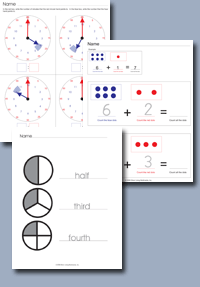Click on any of the standards on the left to create custom worksheets or to print premade materials.